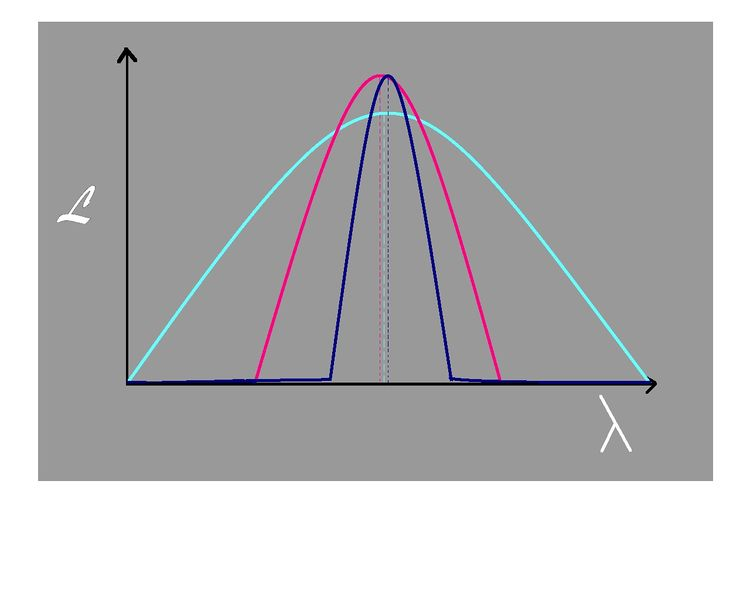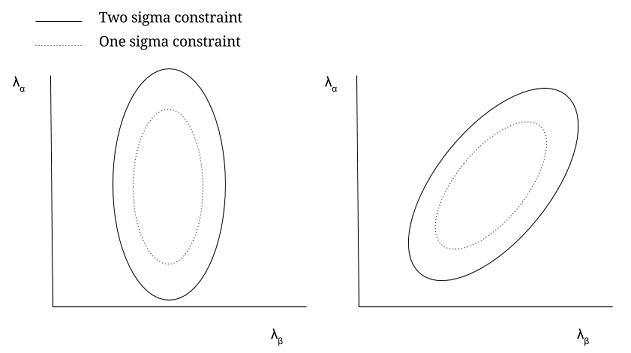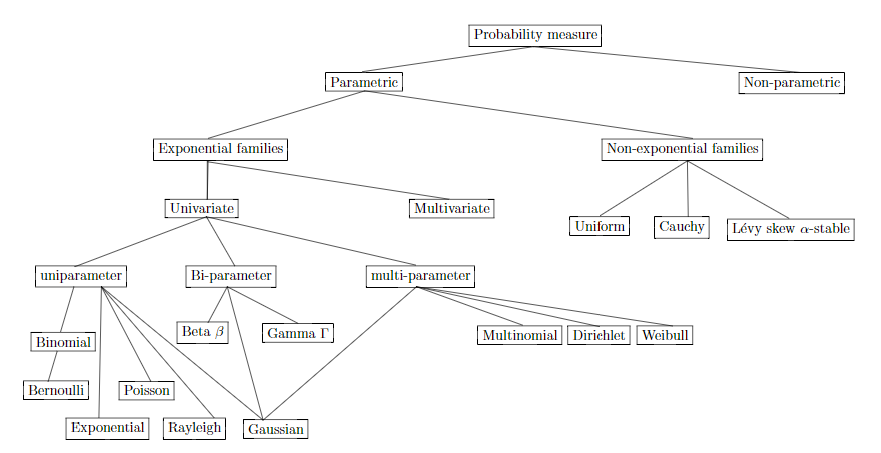### FISHER INFORMATION MATRICES:

Practically transcribed from here, and with modifications from here.

In general, the likelihood function describes the probability of getting a set of data (which will be measured) given an underlying theory by which the universe or what you are measuring can be described (set of parameters):

$\begin{equation} \mathcal{L} \equiv \Pr[\text{data | theory}] \end{equation}$

Below are three examples of likelihood functions (see figure below) – the distribution of probabilities that a particular parameter (here $$\lambda$$) will describe your data. The narrowness of the curve corresponds to how well you will be able to constrain your data. The center value of the very narrow curve is the true value of the parameter–the value at which you are very likely to measure your data set–and the probability of getting your set of measured data falls off as you move away from this true value. Thus, if the value of $$\lambda$$ is equal to this center value, we are far more likely to see the data we measure.However, given a broad distribution like that of the cyan curve, although we are still most likely to recover data at the center $$\lambda$$, there is also a great deal of likelihood we can recover data at values of $$\lambda$$ further away since these still have very high values of $$\mathcal{L}$$. Thus, we are still quite likely to describe the measured data with many values of $$\lambda$$ even though there is a true value.

These differences in the types of curves we can obtain can be quantified and become very useful. To that end, we wish to quantify how quickly the curves fall off from the maximum as a function of the parameter, i.e. the width of the curve. From this, we can ask, given a set of measured data, how constraining are we on the underlying theory–i.e. how well does the data fit the theory? If the curve is narrow (falls off very quickly), the data constrains the theory relatively well, but if the curve is broad (falls off very slowly), the theory is not well constrained by the data.

To quantify this, we Taylor expand the likelihood function around its peak for this simple one-parameter example, $$\lambda_0$$:

$\begin{equation} \mathcal{L} = \mathcal{L}(\lambda_0) + \frac{d \mathcal{L}}{d \lambda} \Bigg|_{\lambda_0} (\lambda - \lambda_0) + {1 \over 2} \frac{d^2 \mathcal{L}}{d \lambda ^2} \Bigg|_{\lambda_0} (\lambda - \lambda_0)^2 + \cdots \end{equation}$

Since we’ve defined $$\lambda_0$$ to be the maximum, the first derivative is equal to zero. Therefore, our first piece of information is in the second derivative of the likelihood function with respect to our parameter. If we neglect terms of higher order than second, we obtain

$\begin{equation} \mathcal{L} \approx \mathcal{L}(\lambda_0) + {1 \over 2} \frac{d^2 \mathcal{L}}{d \lambda ^2} \Bigg|_{\lambda_0} (\lambda - \lambda_0)^2 \end{equation}$

Here, then, we are approximating our likelihood function as a parabola, which is not a good approximation in general since it can have any shape and in fact, even in the simplest cases, the likelihood function is not quadratic in form. A better approximation comes from Taylor expanding the natural log of the likelihood function, which leads to a second derivative that is Gaussian in form ($$\frac{d^2 \ln\mathcal{L}}{d \lambda ^2}$$ for one parameter), which is a far better approximation of a general peak shape (as in those in the above figure).

We can generalize the above to more parameters, and thereby define the Fisher (or curvature) matrix. Here, we define it for the more general case of two parameters

$\begin{equation} \mathcal{F} \equiv - \Bigg \langle \frac{d \ln \mathcal{L}}{d \lambda_{\alpha}} \frac{d \ln \mathcal{L}}{d \lambda_{\beta}} \Bigg \rangle \end{equation}$

where $$\lambda_{\alpha}$$ and $$\lambda_{\beta}$$ are two different parameters we’re using to describe our underlying theory. The Fisher matrix is often called the curvature matrix since it’s the second derivative of the likelihood function, and it indeed describes the curvature of $$\mathcal{L}$$–how quickly it falls off as a function of our parameters. The size of the Fisher matrix values corresponds directly to the shape of the likelihood function–the larger the values, the narrower the curve and the narrower the curve, the more constraining your data is for that parameter and the smaller the uncertainties on the parameter–given by $$\sqrt{\mathcal{F}^{-1}}$$. Thus, the Fisher matrix can be used to forecast how effective an experiment will be in constraining the parameters of an underlying theory; it tells you the best possible constraints you can hope to obtain with a particular experiment and does so by maximizing the likelihood function without the need to cover the whole parameter space.

If Gaussian errors are assumed for each observable, characterized by $$\sigma_l$$, then the elements of the general Fisher matrix (again, assuming Gaussian statistics) are given by

$\begin{equation} \mathcal{F}_{ij} = \sum_l {\frac{1}{\sigma_l^2} \frac{d f_l}{d \lambda_i} \frac{d f_l}{d \lambda_j }} \end{equation}$

where the $$\lambda_i$$ and $$\lambda_j$$ represent N model parameters and there are L observables related to the model parameters by the $$f_l$$ equations, $$f_l = f_l(\lambda_1, \lambda_2, \ldots, \lambda_N)$$.

Another important matrix in statistics is the covariance matrix, and it relates to the Fisher matrix in a very useful way. If we take the inverse of the Fisher matrix ($$\mathcal{F}^{-1}$$), the diagonal elements give us the variance (the square of the uncertainty) of the parameters and the off-diagonal elements are the covariances between the parameters. In particular, the covariance is the degree to which the uncertainty in one parameter affects the uncertainty of another. Therefore, high covariance is not desirable since this can result in errors which are significantly influenced by the covariance when doing the actual data analysis. \ As an explicit example, we can use the above two-parameter ($$\alpha, \beta$$) space. The covariance matrix is given by $\begin{equation} \mathcal{F}_{\alpha \beta}^{-1} = \mathcal{C}_{\alpha \beta} \end{equation}$ where $$\mathcal{C}_{\alpha \beta}$$ is the covariance matrix. Writing this more explicitly, we have $\begin{equation} \mathcal{C}_{\alpha \beta} = \begin{bmatrix} \sigma_{\alpha}^2 & \sigma_{\beta \alpha} \\ \sigma_{\alpha \beta} & \sigma_{\beta}^2 \end{bmatrix} \end{equation}$

where the diagonal elements are the variance of the parameters, which have the conventional statistical definition–they give the spread around the true value (that of the peak)–the off-diagonal are the covariances (the degree to which $$\alpha$$ and $$\beta$$ covary with respect to each other), and the $$\sigma$$ are the uncertainties. \ In the below figure (Figure ), we have two types of contour plots, one with no covariance and one with covariance. In the first figure, there is no covariance–that is, we can take a step in $$\beta$$ without having to change $$\alpha$$ at all. And in the second, there is covariance–each step in $$\alpha$$ or $$\beta$$ forces a change in the other parameter in order to remain consistent with the data. \In the first figure, there is no covariance. In the second figure, the variance is defined in the same way, but now the covariance is nonzero.

How do we make these plots? First, we need to develop a fiducial model. This model uses your best guess values for the true parameter values. In our two-parameter case, we guess a value for $$\lambda_{\alpha}$$ and $$\lambda_{\beta}$$ and this set becomes the center of the contours. Keep in mind then that your Fisher matrix is valid only for models near the fiducial model.

Next, 1- and 2-sigma are defined by the chi-squared distribution (again, this assumes Gaussian statistics!), so from this we can calculate the contours. We calculate chi-squared directly from the Fisher matrix. (This reinforces the usefulness of the Fisher matrix in predicting how well a given experiment or multiple experiments will constrain the parameters of a theory.) The chi-squared is given by $\begin{equation} \chi^2 = \delta \mathcal{F} \delta^T \end{equation}$

where $$\mathcal{F}$$ is the Fisher matrix and $$\delta$$ is some small step away from our fiducial $$\lambda_{\alpha}$$ and $$\lambda_{\beta}$$. From here, we can do a brute force computation See Numerical Recipes or Carl Heiles’ handbook with our Fisher matrix to get the contours. Depending on the number of parameters you have in your model, there are also well defined $$\Delta \chi^2$$ corresponding to $$1-, 2-, 3-\sigma,$$ etc. contours (See Numerical Recipes). An important feature to note in these contour plots is there dependence on the Fisher matrix, namely that the larger the Fisher matrix element values are (corresponding to narrower likelihood curves), the smaller the covariance and therefore variance, and thus, the smaller the contours become. Therefore, with larger Fisher matrices, we get smaller contours and thus, parameters that are better constrained, which is exactly what we want! For examples of calculating the chi-squared and Fisher matrix see Jonathan Pober’s. An important thing to note in all of this as well is that when calculating the Fisher matrix, it has no dependence on the observed data–only on how the parameters are related to the model–so we can determine the validity of experiments and how well a theory can be constrained before we ever observe–saving time and resources!

From here:

Fisher Information is the KL divergence between a model estimate and an infinitesimal change in that estimate. It is also inversely proportional to the error rate between. Therefore, it is a extremely important metric on what model parameters to adjust to improve one’s model fitting. Shannon entropy is a global estimate of a probability distribution as compared to Fisher Information which is a local estimate.

For any random variable $$X$$ with finite variance, we have that $$\frac{\partial}{\partial t}h_e\left(X + \sqrt{t}Z\right) = \frac{1}{2}J\left(X + \sqrt{t}Z\right)$$ where $$h_e$$ is the differential entropy with base $$e$$, $$J$$ is the Fisher information, and $$Z$$ is a standard normal random variable which is independent of $$X$$.

#### Exponential family of distributions:

From this source:

An exponential family is a set of probability distributions admitting the following canonical decom-position:

$p(x;θ) = \exp (\left < t(x), \theta\right>−F(\theta) +k(x))$

where $$t(x)$$ is the sufficient statistic. The sufficient statistic $$t(x)$$ should be elementary functions (often polynomial functions) with leading unit coefficient. $$\theta$$ are the natural parameters, $$\left<\;,\;\right>$$ is the inner product (commonly called dot product), $$F(\cdot)$$ is the log-normalizer

$$F(\theta) = \log \int_x \exp(\left< t(x), \theta \right> +k(x))dx,$$

and $$k(x)$$ is the carrier measure. The carrier measure should not have constant term, so that we prefer to ab-sorb the constant in the log-normalizer.

Exponential families include many familiar distributions, characterized by their log-normaliser functions:

Gaussian or normal (generic, isotropic Gaussian, diagonal Gaussian, rectified Gaussian or Wald distributions, log-normal), Poisson, Bernoulli, binomial, multinomial (trinomial, Hardy-Weinberg distribution), Laplacian, Gamma (including the chi-squared), Beta, exponential, Wishart, Dirich-let, Rayleigh, probability simplex, negative binomial distribution, Weibull, Fisher-von Mises, Pareto distributions, skew logistic, hyperbolic secant, negative binomial, etc.For exponential families, we have

$$E[t(X)]=\mu=\nabla F(\theta)$$

$$E[(t(X)-\mu)^\top(t(X)-\mu)]=\nabla^2F(\theta)$$

$$\nabla^2 F$$ denotes the Hessian of the log-normalizer. It is a positive definite matrix since $$F$$ is strictly convex and differentiable function. In fact, exponential families have all finite moments, and $$F$$ is $$C^\infty$$ differentiable.

The natural parameter space $$\mathcal P = \{\Theta | F(\Theta)| < +\infty\}$$ is necessarily an open convex set.

A family of parametric distributions $$\{p(x;\theta)\}$$ (exponential or not) may be thought as a smooth manifold that can be studied using the framework of differential geometry. Two main types of geometries: (1) Riemannian geometry defined by a bilinear tensor with an induced Levi-Cevita connection, and non-metric geometry induced by a symmetric affine connection.

the only Riemannian metric that “makes sense” for statistical manifolds is the Fisher information metric:

$I(\theta) = \left [\int \frac{\partial \log p(x;\theta)}{\partial \theta_i} \frac{\partial \log p(x;\theta)}{\partial \theta_j} p(x; \theta)\;dx\right ]= [g_{ij}]$ The infinitesimal length element is given by

$\mathrm d s^2 = \sum_{i=1}^d\sum_{i=1}^d \mathrm d\theta_i^\top \nabla^2F(\theta)\mathrm \theta_j$

Cencov proved that for a non-singular transformation of the parameters $$\lambda=f(\theta),$$ the information matrix

$I(\lambda)=\left[ \frac{\partial \theta_i}{\partial \lambda_j} \right]\quad I(\theta)=\left[ \frac{\partial \theta_i}{\partial \lambda_j} \right]$

is such that $$\mathrm ds^2(\lambda)=\mathrm d s^2(\theta).$$

Equipped with the tensor $$I(\theta)$$, the metric distance between two distributions on a statistical manifold can be computed from the geodesic length (e.g., shortest path):

$D\,\left ( p(x;\theta_1)\,,\,p(x;\theta_2) \right)=\min_{\theta(t)\,|\,\theta(0)=\theta_1\;,\;\theta(1)=\theta_2}\;\int_0^1 \; \sqrt{\left(\frac{\mathrm d\theta}{\mathrm dt} \right)^\top\;I(\theta)\frac{\mathrm d \theta}{\mathrm dt}dt}$

The multinomial Fisher-Rao-Riemannian geometry yields a spherical geometry, and the normal Fisher-Rao-Riemannian geometry yields a hyperbolic geometry. Indeed, the Fisher information matrix for univariate normal distributions is

$I(\theta) = \frac{1}{\sigma^2}\begin{bmatrix}1&0\\0&2 \end{bmatrix}$

The Fisher information matrix can be interpreted as the Hessian of the Shannon entropy:

$g_{ij}(\theta)=-E\left[ \frac{\partial^2\log p(x;\theta)}{\partial \theta_i \partial\theta_j} \right]=\frac{\partial^2 H(p)}{\partial \theta_i \partial \theta_j}$

with $H(p) = -\int p(x;\theta)\,\log p(x;\theta) \mathrm dx.$

For an exponential family, the Kullback-Leibler divergence is a Bregman divergence on the natural parameters. Using Taylor approximation with exact remainder, we get

$\mathrm {KL}(\theta||\theta + \mathrm d\theta) = \frac{1}{2} \mathrm d\theta^\top \nabla^2\,F(θ)\mathrm d\theta.$

Moreover, the infinitesimal Rao distance is $\sqrt{\mathrm d\theta^T I(\theta)\mathrm d\theta}$ for $$I(\theta) = \nabla^2 F(\theta).$$ We deduce that $(, + d) = .$

The inner product of two vectors $$\vec x$$ and $$\vec y$$ at a tangent space of point $$p$$ is

$\left< x,y \right >_p=\vec x^\top\, g_p \vec y.$

Technically speaking, it is a bilinear symmetric positive definite oprator. A tensor $$[T_p]^2_2$$ of covariant degree $$2$$ and a contravariant degree $$0: <\cdot,\cdot>_p: T_p \times T_p \to \mathbb R.$$

The length of the vector $$\vec v$$ in the tangent space at $$T_p$$ at $$p$$ is defined by $$\Vert \vec v \Vert_p = \sqrt{\left<v,v \right>_p}$$

For exponential families, the logarithm of the density is concave (since $$F$$ is convex), and we have

$I(\theta)=\left[ \frac{\partial \eta}{\partial \theta}\right]=\nabla^2F(\theta)=I^{-1}(\eta)=\left[\frac{\partial\theta}{\partial \eta} \right]$ That is, the Fisher information is the Hessian of the log-normalizer $$\nabla^2 F(\theta).$$

To a given manifold $$\mathcal M$$ with tensor $$G,$$ we may associeate a probability measure as follows: Let $$p(\theta)=\frac{1}{V}\sqrt{\det G(\theta)}$$ with overall volume $$V=\int_{\theta\in \Theta}\sqrt{G(\theta)}\mathrm d\theta.$$ These distributions are built from infinetisimal volume elements and bear the name of Jeffreys priors. They are used in Bayesisan estimation.

Furthermore, in an exponential family manifold, the geometry is flat and $$\theta/\eta$$ are dual coordinate systems.

Amari focused on a pair of dual affine mixture/exponential connections $$\nabla^m$$ and $$\nabla^e$$ induced by a contrast function $$F$$ (also called potential function). A connection $$\nabla$$ yields a function $$\Pi_{p,q}$$ that maps vectors in any pair of tangent spaces $$T_p$$ and $$T_q.$$ An affine connection is defined by $$d^3$$ coefficients. $$\nabla^{(0)}$$, the metric Levi-Civita connection, is obtained from the dual $$\nabla^{(\alpha)}$$ and $$\nabla^{(-\alpha)}$$ $$\alpha$$-connections:

$\nabla^{(0)}=\frac{\nabla^{(\alpha)}+\nabla^{(-\alpha)}}{2}.$

In particular, the $$\nabla^{(1)}$$ connection is called the exponential connection and the $$\nabla^{(-1)}$$ connection is called the mixture connection. The mixture/exponential geodesics for the two distributions $$p(x)$$ and $$q(x)$$ induced by these dual connections are defined by

$\gamma_\lambda(x)=(1-\lambda)p(x)+\lambda q(x)$

$\log_{\gamma_\lambda}(x) = (1-\lambda)\log p(x) + \lambda \log q(x) - \log Z_\lambda$

with $$Z_\lambda$$ the normalization coefficient $$Z_\lambda =\int f^{(1-\lambda)}(x)g^\lambda(x) dx.$$

#### Appendix:

In information geometry, the Fisher information metric is a particular Riemannian metric which can be defined on a smooth statistical manifold, i.e., a smooth manifold whose points are probability measures defined on a common probability space. It can be used to calculate the informational difference between measurements.

It can be understood to be the infinitesimal form of the relative entropy (i.e., the Kullback–Leibler divergence); specifically, it is the Hessian of the divergence. In turn, the Kullback-Leibler divergence is a generalization of the Mahalanobis distance.

In mathematical statistics, the Kullback–Leibler divergence (also called relative entropy) is a measure of how one probability distribution diverges from a second, expected probability distribution.

For distributions $$P$$ and $$Q$$ of a continuous random variable, the Kullback–Leibler divergence is defined to be the integral:

$D_\mathrm {KL}(P||Q) = \int_{-\infty}^\infty p(x)\log\frac{p(x)}{q(x)} dx$

which amounts to the expectation of the logarithmic difference between the probabilities $$P$$ and $$Q.$$ Actually, $$\frac{p(x)}{q(x)}$$ is the likelihood ratio.